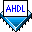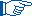## Declaring Registers

 See AlsoRegisters store data values and synchronize data with a clock signal. You can declare, that is, implement, an instance of a register with a Register Declaration in the Variable Section. (You can also implement registers with in-line logic function references in the Logic Section.) AHDL offers several register primitives and also supports registered library of parameterized modules (LPM) functions.

Once you have declared a register, you can connect it to other logic in the Text Design File (.tdf) by using its ports. A port of an instance is used in the following format:

<instance name>`.`<port name>

The bur_reg.tdf file shown below uses a Register Declaration to create a byte register that latches values of the `d` inputs onto the `q` outputs on the rising edge of the clock when the `load` input is high.

```SUBDESIGN bur_reg
(
q[7..0]            : OUTPUT;
)
VARIABLE
ff[7..0]           : DFFE;
BEGIN
ff[].clk = clk;
ff[].d = d[];
q[] = ff[].q;
END;
```

The registers are declared as enable D flipflops (`DFFE`) in the Variable Section. The first Boolean equation in the Logic Section connects the bur_reg.tdf file's clock input, `clk`, to the clock ports of the `ff[7..0]` flipflops. The second equation connects the `load` input to the clock enable ports. The third equation connects the file's data inputs, `d[7..0]`, to the data input ports of the `ff[7..0]` flipflops. The fourth equation connects the file's outputs to the flipflop outputs. All four statements are evaluated concurrently.

You can also declare T, JK, and SR flipflops in the Variable Section, and then use them in the Logic Section. For example, for T flipflops (`TFF`), you would change the Register Declaration to `ff[7..0] : TFF;` and change `ff[].d` to `ff[].t` in the third equation. Similarly, for enable JK flipflops (`JKFFE`), you would change the Register Declaration to `ff[7..0] : JKFFE;` and replace the third equation with two equations that connect the `ff[].j` and `ff[].k` ports to other signals.If you wish to load a register on a specific rising edge of the global clock, Altera® recommends that you use the clock enable input of one of the `DFFEA`, `DFFE`, `TFFE`, `JKFFE`, or `SRFFE` enable-type flipflops to control when the register is loaded.

The lpm_reg.tdf file shown below uses an in-line logic function reference to implement an instance of the `lpm_dff` function that has the same functionality as the bur_reg.tdf file.

```INCLUDE "lpm_dff.inc";
SUBDESIGN lpm_reg
(
q[7..0]            : OUTPUT;
)
BEGIN
q[] = lpm_dff (.clock=clk, .enable=load, .data[]=d[])
WITH (LPM_WIDTH=8)
RETURNS (.q[]);
END;
```